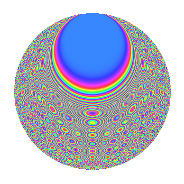# Properties

 Label 2006.2.gLevel 2006 Weight 2 Character orbit g Rep. character $$\chi_{2006}(355,\cdot)$$ Character field $$\Q(\zeta_{8})$$ Dimension 352 Sturm bound 540

# Related objects

## Defining parameters

 Level: $$N$$ = $$2006 = 2 \cdot 17 \cdot 59$$ Weight: $$k$$ = $$2$$ Character orbit: $$[\chi]$$ = 2006.g (of order $$8$$ and degree $$4$$) Character conductor: $$\operatorname{cond}(\chi)$$ = $$17$$ Character field: $$\Q(\zeta_{8})$$ Sturm bound: $$540$$

## Dimensions

The following table gives the dimensions of various subspaces of $$M_{2}(2006, [\chi])$$.

Total New Old
Modular forms 1096 352 744
Cusp forms 1064 352 712
Eisenstein series 32 0 32

## Trace form

 $$352q + 16q^{5} + O(q^{10})$$ $$352q + 16q^{5} + 16q^{10} - 16q^{11} + 8q^{15} - 352q^{16} - 32q^{18} - 32q^{25} + 16q^{26} + 48q^{27} + 16q^{28} - 16q^{29} + 32q^{31} + 64q^{33} + 32q^{35} + 32q^{37} - 32q^{39} - 16q^{41} - 48q^{42} - 32q^{43} - 32q^{44} + 24q^{45} + 32q^{46} + 80q^{49} - 32q^{51} - 32q^{52} - 32q^{53} - 8q^{60} - 16q^{61} + 40q^{63} - 32q^{65} + 32q^{67} - 64q^{69} + 32q^{70} + 64q^{71} - 64q^{73} - 16q^{74} + 88q^{75} + 16q^{77} - 16q^{80} - 64q^{82} - 16q^{83} + 112q^{85} + 48q^{86} - 32q^{88} - 96q^{91} - 16q^{92} + 144q^{93} + 48q^{99} + O(q^{100})$$

## Decomposition of $$S_{2}^{\mathrm{new}}(2006, [\chi])$$ into irreducible Hecke orbits

The newforms in this space have not yet been added to the LMFDB.

## Decomposition of $$S_{2}^{\mathrm{old}}(2006, [\chi])$$ into lower level spaces

$$S_{2}^{\mathrm{old}}(2006, [\chi]) \cong$$ $$S_{2}^{\mathrm{new}}(17, [\chi])$$$$^{\oplus 4}$$$$\oplus$$$$S_{2}^{\mathrm{new}}(34, [\chi])$$$$^{\oplus 2}$$$$\oplus$$$$S_{2}^{\mathrm{new}}(1003, [\chi])$$$$^{\oplus 2}$$